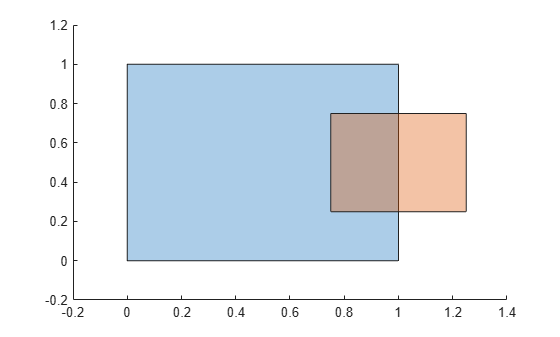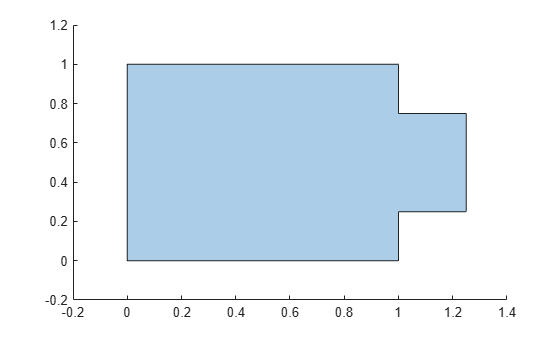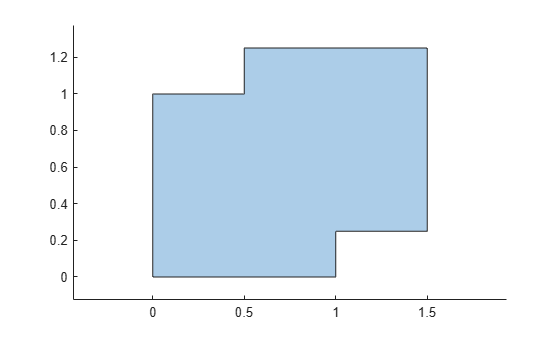# union

Union of `polyshape` objects

## Syntax

``polyout = union(poly1,poly2)``
``polyout = union(polyvec)``
``[polyout,shapeID,vertexID] = union(poly1,poly2)``
``[polyout,shapeID,vertexID] = union(polyvec)``
``___ = union(___,'KeepCollinearPoints',TF)``

## Description

example

````polyout = union(poly1,poly2)` returns a `polyshape` object whose regions are the union of two `polyshape` objects. The union contains the combined regions of `poly1` and `poly2`, which must have compatible array sizes.```

example

````polyout = union(polyvec)` returns a `polyshape` object whose regions are the geometric union of all `polyshape` objects in the vector `polyvec`. The union contains the combined regions of the `polyshape` objects in `polyvec`.```

example

````[polyout,shapeID,vertexID] = union(poly1,poly2)` also returns vertex mapping information from the vertices in `polyout` to the vertices in `poly1` and `poly2`. The `union` function only supports this syntax when `poly1` and `poly2` are scalar `polyshape` objects.The `shapeID` elements identify whether the corresponding vertex in `polyout` originated in `poly1`, `poly2`, or was created from the union. `vertexID` maps the vertices of `polyout` to the vertices of `poly1`, `poly2`, or the union.```
````[polyout,shapeID,vertexID] = union(polyvec)` returns vertex mapping information from `polyvec` to each element of the vector of `polyshape` objects `polyvec`.```
````___ = union(___,'KeepCollinearPoints',TF)` specifies whether to keep or remove collinear points in `polyout` for any of the previous syntaxes.```

## Examples

collapse all

Create and plot two polygons.

```poly1 = polyshape([0 0 1 1],[1 0 0 1]); poly2 = polyshape([0.75 1.25 1.25 0.75],[0.25 0.25 0.75 0.75]); plot(poly1) hold on plot(poly2)````figure`

Compute and plot the union of `poly1` and `poly2`.

`polyout = union(poly1,poly2)`
```polyout = polyshape with properties: Vertices: [8x2 double] NumRegions: 1 NumHoles: 0 ```
```plot(polyout) xlim([-0.2 1.4]); ylim([-0.2 1.2]);```Create a vector of polygons and plot each polygon.

```polyarray1 = polyshape([0 0 1 1],[1 0 0 1]); polyarray2 = polyshape([0.75 1.25 1.25 0.75],[0.25 0.25 0.75 0.75]); poly1 = [polyarray1 polyarray2]```
```poly1 = 1x2 polyshape array with properties: Vertices NumRegions NumHoles ```
```plot(poly1(1)) hold on plot(poly1(2))````figure`

Compute and plot the union of the two polygons.

`polyout = union(poly1)`
```polyout = polyshape with properties: Vertices: [8x2 double] NumRegions: 1 NumHoles: 0 ```
```plot(polyout) xlim([-0.2 1.4]); ylim([-0.2 1.2]);```Create two polygons, and compute and plot their union. Display the vertex coordinates of the union and the corresponding vertex mapping information.

```poly1 = polyshape([0 0 1 1],[1 0 0 1]); poly2 = translate(poly1,[0.5 0]); [polyout,shapeID,vertexID] = union(poly1,poly2); plot(polyout) axis equal````[polyout.Vertices shapeID vertexID]`
```ans = 4×4 0 1.0000 1.0000 1.0000 1.5000 1.0000 2.0000 2.0000 1.5000 0 2.0000 3.0000 0 0 1.0000 4.0000 ```

The first and last vertices of the union originated in `poly1`, since the corresponding values in `shapeID` are 1. These vertices are the first and fourth vertices in the property `poly1.Vertices`, respectively, since the corresponding values in `vertexID` are 1 and 4. Similarly, the second and third vertices of the union originated in `poly2`, and they are the second and third vertices in the property `poly2.Vertices`, respectively.

## Input Arguments

collapse all

First input `polyshape`, specified as a scalar, vector, matrix, or multidimensional array.

Second input `polyshape`, specified as a scalar, vector, matrix, or multidimensional array.

`polyshape` vector.

Collinear vertices indicator, specified as `false` or `true`:

• `false` — Remove collinear points so that the output `polyshape` contains the fewest vertices necessary to define the boundaries.

• `true` — Keep all collinear points as vertices.

When the `'KeepCollinearPoints'` parameter is not specified, its value is assigned according to the values used when creating the input `polyshape` objects:

• If the value was `true` for each input `polyshape` when they were created, then the value for the output `polyshape` is set to `true`.

• If the value was `false` for each input `polyshape` when they were created, then the value for the output `polyshape` is set to `false`.

• If the values for the input `polyshape` objects do not match, then the value for the output `polyshape` is set to `false`.

Data Types: `logical`

## Output Arguments

collapse all

Output `polyshape`, returned as a scalar, vector, matrix, or multidimensional array.

• If you input two `polyshape` arguments, then they must have compatible sizes. For example, if two input `polyshape` vectors have different lengths M and N, then they must have different orientations (one must be a row vector and one must be a column vector). `polyout` is then M-by-N or N-by-M depending on the orientation of each input vector. For more information on compatible array sizes, see Compatible Array Sizes for Basic Operations.

• If you provide a single input argument `polyvec`, then `polyout` is a scalar `polyshape` object.

Shape ID, returned as a column vector whose elements each represent the origin of the vertex in the union.

• The length of `shapeID` is equal to the number of rows in the `Vertices` property of the output `polyshape`.

• The elements of `shapeID` depend on the number of input arguments:

• If you provide two input arguments `poly1` and `poly2`, then they must be scalar `polyshape` objects. The value of an element in `shapeID` is 0 when the corresponding vertex of the output `polyshape` was created by the union. An element is 1 when the corresponding vertex originated from `poly1`, and 2 when it originated from `poly2`.

• If you provide one input argument `polyvec` that is a vector of `polyshape` objects, then `shapeID` contains the element index of `polyvec` from which the corresponding output vertex originated. The value of an element is 0 when the corresponding vertex was created by the union.

Data Types: `double`

Vertex ID, returned as a column vector whose elements map the vertices in the output `polyshape` to the vertices in the `polyshape` of origin. The elements of `vertexID` contain the row numbers of the corresponding vertices in the `Vertices` property of the input `polyshape`. An element is 0 when the corresponding vertex of the output `polyshape` was created by the union.

The length of `vertexID` is equal to the number of rows in the `Vertices` property of the output `polyshape`. If you provide two input `polyshape` objects, then `union` only supports this output argument if they are scalar.

Data Types: `double`